CBSE Physics Sample Papers and Syllabus for Class 12th

CBSE 10th Date sheet 2021      CBSE 12th Date Sheet 2021

SECTION A

This section consists of 25 multiple choice questions with overall choice to attempt any 20 questions. In case more than desirable numberof questions are attempted, ONLY first 20 will be considered for evaluation.

1. Which of the following is NOT the property of equipotential surface?

• They do not cross each other.
• The rate of change of potential with distance on them is zero.
• For a uniform electric field they are concentric spheres.
• They can be imaginary spheres.

• 8L
• 4L
• 2 L
• L

• 2pE
• pE
• pE/2
• Zero

• 1/2µF
• 1µF
• 2µF
• 11µF

• 5F
• F
• F/2
• F/5

6. Which statement is true for Gauss law-

• All the charges whether inside or outside the gaussian surface contribute to the electric flux.
• Electric flux depends upon the geometry of the gaussian surface.
• Gauss theorem can be applied to non-uniform electric field.
• The electric field over the gaussian surface remains continuous and uniform at every point.

• Zero
• V/2
• V/K
• KV

8. The best instrument for accurate measurement of EMF of a cell is-

• Potentiometer
• metre bridge
• Voltmeter
• ammeter and voltmeter

• 2:3
• 3:2
• 8:27
• 27:8

10. By increasing the temperature, the specific resistance of a conductor and a semiconductor-

• increases for both.
• decreases for both.
• increases for a conductor and decreases for a semiconductor.
• decreases for a conductor and increases for a semiconductor.

11. We use alloys for making standard resistors because they have

• low temperature coefficient of resistivity and high specific resistance
• high temperature coefficient of resistivity and low specific resistance
• low temperature coefficient of resistivity and low specific resistance
• high temperature coefficient of resistivity and high specific resistance

• H/2
• H
• 2H
• 4H

13. If the potential difference V applied across a conductor is increased to 2V with its temperature kept constant the drift velocity of the free electrons in a conductor will -

• remain the same
• become half of its previous value
• be double of its initial value
• become zero.

14. The equivalent resistance between A and B is-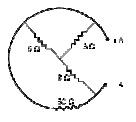• 3 ohms
• 5.5 ohms
• 7.5 ohms
• 9.5 ohms

• AmN-1
• NA-1m-1
• NA-2m-2
• NA-1m-2

16. The coil of a moving coil galvanometer is wound over a metal frame in order to

• reduce hysteresis
• increase sensitivity
• increase moment of inertia
• provide electromagnetic damping

• 2 : π
• π : 2
• π : 4
• 4 : π

• π/6
• π/3
• π/4
• 0

19. The small angle between magnetic axis and geographic axis at a place is-

• Magnetic meridian
• Geographic meridian
• Magnetic inclination
• Magnetic Declination

20. Two coils are placed close to each other. The mutual inductance of the pair of coils depends upon the

• rate at which current change in the two coils
• relative position and orientation of the coils
• rate at which voltage induced across two coils
• currents in the two coils

21. A conducting square loop of side 'L' and resistance 'R' moves in its plane with the uniform velocity 'v' perpendicular to one of its sides. A magnetic induction 'B' constant in time and space pointing perpendicular and into the plane of the loop exists everywhere as shown in the figure. The current induced in the loop is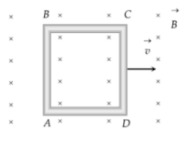• BLv/R Clockwise
• BLv/R Anticlockwise
• 2BLv/R Anticlockwise
• Zero

• -27 V
• -43 V
• -108 V
• 210 V

23. Which of the following graphs represent the variation of current(I) with frequency (f) in an AC circuit containing a pure capacitor?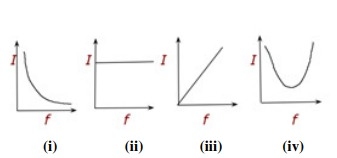• 16 V
• 10 V
• 8 V
• 6 V

25. The instantaneous values of emf and the current in a series ac circuit are- E = Eo Sin ωt and I= Io sin( ωt+π/3) respectively, then it is

• Necessarily a RL circuit
• Necessarily a RC circuit
• Necessarily a LCR circuit
• Can be RC or LCR circuit

The correct answer is Can be RC or LCR circuit.

SECTION B

This section consists of 24 multiple choice questions with overall choice to attempt any 20 questions. In case more than desirable number of questions are attempted, ONLY first 20 will be considered for evaluation.

• zero
• π r2
• E π r2
• 2 Eπ r2

27. Two parallel large thin metal sheets have equal surface densities 26.4x10-12 C/m2 of opposite signs. The electric field between these sheets is-

• 1.5N/C
• 1.5 x 10-16 N/C
• 3 x 10-10N/C
• 3N/C

28. Consider an uncharged conducting sphere. A positive point charge is placed outside the sphere. The net charge on the sphere is then,

• negative and uniformly distributed over the surface of sphere
• positive and uniformly distributed over the surface of sphere
• negative and appears at a point the surface of sphere closest to point charge.
• Zero

• E#0 and V#0
• E=0 and V=0
• E#0 and V=0
• E=0 and V#0

• 4:1
• 1:4
• 2:1
• 1:2

31. Which among the following, is not a cause for power loss in a transformer-

• Eddy currents are produced in the soft iron core of a transformer.
• Electric Flux sharing is not properly done in primary and secondary coils.
• Humming sound produed in the tranformers due to magnetostriction.
• Primary coil is made up of a very thick copper wire.

32. An alternating voltage source of variable angular frequency ‘w’ and fixed amplitude ‘V’ is connected in series with a capacitance C and electric bulb of resistance R(inductance zero). When ‘w’ is increased-

• The bulb glows dimmer.
• The bulb glows brighter.
• Net impedance of the circuit remains unchanged.
• Total impedance of the circuit increases.

33. A solid spherical conductor has charge +Q and radius R. It is surrounded by a solid spherical shell with charge -Q, innerradius 2R, and outer radius 3R. Which of the following statements is true?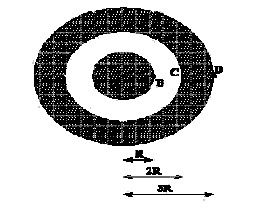• The electric potential has a maximum magnitude at C and the electric field has a maximum magnitude at A
• The electric potential has a maximum magnitude at D and the electric field has a maximum magnitude at B.
• The electric potential at A is zero and the electric field has a maximum magnitude at D.
• Both the electric potential and electric field achieve a maximum magnitude at B.

34. A battery is connected to the conductor of non-uniform cross section area. The quantities or quantity which remains constant is-

• electric field only
• drift speed and electric field
• electric field and current
• current only

• 1V
• 2V
• 3V
• 4V

• 2.8 ohms
• 1.4 ohms
• 1.2 ohms
• zero

37. A 10 m long wire of uniform cross-section and 20 Ω resistance is used in a potentiometer. The wire is connected in series with a battery of 5 V along with an external resistance of 480 Ω. If an unknown emf E is balanced at 6.0 m length of the wire then the value of unknown emf is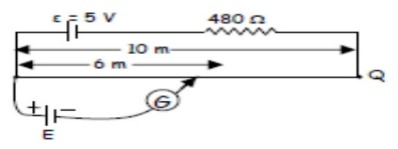• 1.2 V
• 1.02 V
• 0.2 V
• 0.12 V

38. The current sensitivity of a galvanometer increases by 20%. If its resistance also increases by 25%, the voltage sensitivity will

• decrease by 1%
• increased by 5%
• increased by 10%
• decrease by 4%

39. Three infinitely long parallel straight current carrying wires A, B and C are kept at equal distance from each other as shown in the figure . The wire C experiences net force F .The net force on wire C, when the current in wire A is reversed will be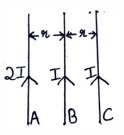• Zero
• F/2
• F
• 2F

40. In a hydrogen atom the electron moves in an orbit of radius 0.5 Ao making 10 revolutions per second, the magnetic moment associated with the orbital motion of the electron will be

• 2.512 x 10-38 Am2
• 1.256 x 10-38 Am2
• 0.628 X10-38 Am2
• zero

• zero
• 3.125 volts
• 6.54 volts
• 16.74 volts

• 39.70 kW
• 26.70 kW
• 13.35 kW
• 13.35 kW

43. A circular loop of radius 0.3cm lies parallel to much bigger circular of radius 20 cm. The centre of the small loop is on the axis of the bigger loop. The distance between their centres is 15 cm. If a current of 2.0 A flows through the smaller loop, then the flux linked with the bigger loop is

• 3.3 X 10-11weber
• 6 X 10-11weber
• 6.6 X 10-9weber
• 9.1 X 10-11weber

• Unaffected
• doubled
• halved

45. Given below are two statements labelled as Assertion (A) and Reason (R)

Assertion (A): To increase the range of an ammeter, we must connect a suitable high resistance in series to it.

Reason (R): The ammeter with increased range should have high resistance.

Select the most appropriate answer from the options given below:

• Both A and R are true and R is the correct explanation of A
• Both A and R are true but R is not the correct explanation of A.
• A is true but R is false.
• A is false and R is also false.

46. Given below are two statements labelled as Assertion (A) and Reason (R)

Assertion (A): An electron has a high potential energy when it is at a location associated with a more negative value of potential, and a low potential energy when at a location associated with a more positive potential.

Reason (R):Electrons move from a region of higher potential to region of lower potential.

Select the most appropriate answer from the options given below:

• Both A and R are true and R is the correct explanation of A
• Both A and R are true but R is not the correct explanation of A.
• A is true but R is false.
• A is false and R is also false.

47. Given below are two statements labelled as Assertion (A) and Reason (R)

Assertion(A): A magnetic needle free to rotate in a vertical plane, orients itself (with its axis) vertical at the poles of the earth.

Reason (R): At the poles of the earth the horizontal component of earth’s magnetic field will be zero.

Select the most appropriate answer from the options given below:

• Both A and R are true and R is the correct explanation of A
• Both A and R are true but R is not the correct explanation of A.
• A is true but R is false.
• A is false and R is also false.

48. Given below are two statements labelled as Assertion (A) and Reason (R)

Assertion(A): A proton and an electron, with same momenta, enter in a magnetic field in a direction at right angles to the lines of the force. The radius of the paths followed by them will be same.

Reason(R): Electron has less mass than the proton.

Select the most appropriate answer from the options given below:

• Both A and R are true and R is the correct explanation of A
• Both A and R are true but R is not the correct explanation of A.
• A is true but R is false.
• A is false and R is also false.

49. Given below are two statements labelled as Assertion (A) and Reason (R)

Assertion (A):On Increasing the current sensitivity of a galvanometer by increasing the number of turns, may not necessarily increase its voltage sensitivity.

Reason(R ): The resistance of the coil of the galvanometer increases on increasing the number of turns.

Select the most appropriate answer from the options given below:

• Both A and R are true and R is the correct explanation of A
• Both A and R are true but R is not the correct explanation of A.
• A is true but R is false.
• A is false and R is also false.

The correct answer is Both A and R are true and R is the correct explanation of A

SECTION C

This section consists of 6 multiple choice questions with an overall choice to attempt any 5. In case more than desirable number of questions are attempted, ONLY first 5 will be considered for evaluation.

50. A small object with charge q and weight mg is attached to one end of a string of length ‘L’ attached to a stationary support. The system is placed in a uniform horizontal electric field ‘E’, as shown in the accompanying figure. In the presence of the field, the string makes a constant angle θ with the vertical. The sign and magnitude of q-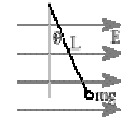• positive with magnitude mg/E
• positive with magnitude (mg/E)tanθ
• negative with magnitude mg/E tanθ
• positive with magnitude E tanθ/mg

51. A free electron and a free proton are placed between two oppositely charged parallel plates. Both are closer to the positive plate than the negative plate.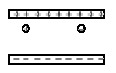Which of the following statements is true?

1. The force on the proton is greater than the force on the electron.
2. The potential energy of the proton is greater than that of the electron.
3. The potential energy of the proton and the electron is the same.
• I only
• II only
• III and I only
• II and I only

The correct answer is II only

Case study :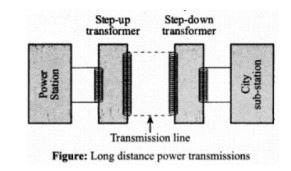The large-scale transmission and distribution of electrical energy over long distances is done with the use of transformers. The voltage output of the generator is stepped-up. It is then transmitted over long distances to an area sub-station near the consumers. There the voltage is stepped down. It is further stepped down at distributing sub-stations and utility poles before a power supply of 240 V reaches our homes.

52. Which of the following statement is true?

• Energy is created when a transformer steps up the voltage
• A transformer is designed to convert an AC voltage to DC voltage
• Step–up transformer increases the power for transmission
• Step–down transformer decreases the AC voltage

53. If the secondary coil has a greater number of turns than the primary

• the voltage is stepped-up (Vs >Vp ) and arrangement is called a step-up transformer
• the voltage is stepped-down (Vs Vp ) and arrangement is called a step- down transformer
• the current is stepped-up (Is >Ip ) and arrangement is called a step-up transformer
• the current is stepped-down (Is Ip ) and arrangement is called a step- down transformer

54. We need to step-up the voltage for power transmission, so that

• the current is reduced and consequently, the I 2R loss is cut down
• the voltage is increased , the power losses are also increased
• the power is increased before transmission is done
• the voltage is decreased so V2 /R losses are reduced

55. A power transmission line feeds input power at 2300 V to a step down transformer with its primary windings having 4000 turns. The number of turns in the secondary in order to get output power at 230 V are?

• 4
• 40
• 400
• 4000Know about the foods that trigger MigraineHow do our diets affect migraine? Many things can trigger a migraine attack, but what we eat and drink in a day is significant considering their consequences. Ten common food… Read More...Know about the Haritha Haram programme"Nuclear arms and Global warming are the major two threats to the human existence," Noam Chomsky, a World-renowned, living public intellectual, stated in his book named Hegemony or Survival: America's… Read More...Know about the Rythu Bandhu SchemeThe Telangana government launched "Rythu Bandhu" on May 10, 2018. It is a welfare scheme that aims to aid farmers in their investments in cropping seasons. It is one of… Read More...What are the services rendered by an advertising agency?The primary objective of an advertising agency is to ensure that its clients' advertising results have increased earnings in the long term. As a result, the agency creates, prepares, and… Read More...